# Algorithms

An algorithm (also called column algorithm) is a Data Block whose values are calculated with a formula based on other Data Blocks.

The following example is a report with a Layout having three data blocks:

• Block "a" is Gross Sales of the year 2021
• Block "b" is Gross Sales of the previous year
• Block "c" contains an algorithm that calculates the percentage of variation using the formula  (a-b)/b*100.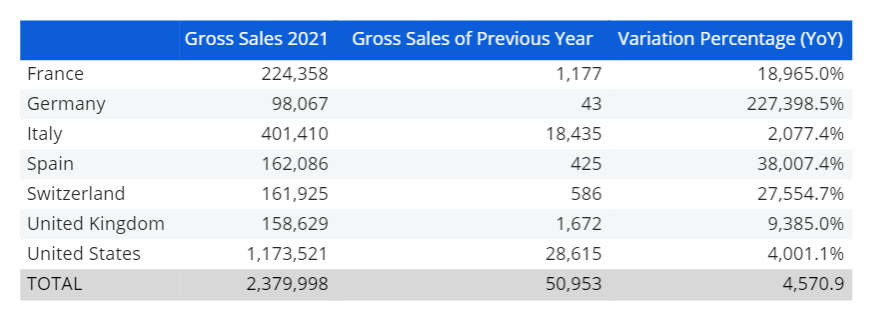Formulas use block letters to refer to other Data Blocks and can also refer to other algorithms. See the table below for a list of supported operators.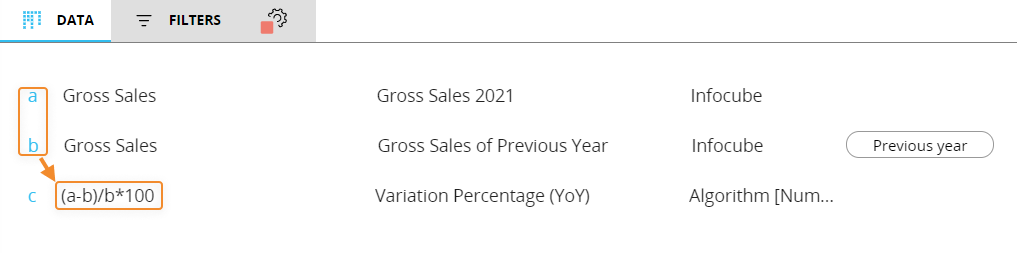A formula can return the following types of data:

• Numeric (default)
• Text
• Date
• Picture

Select the appropriate option from the Algorithm type dropdown list as shown.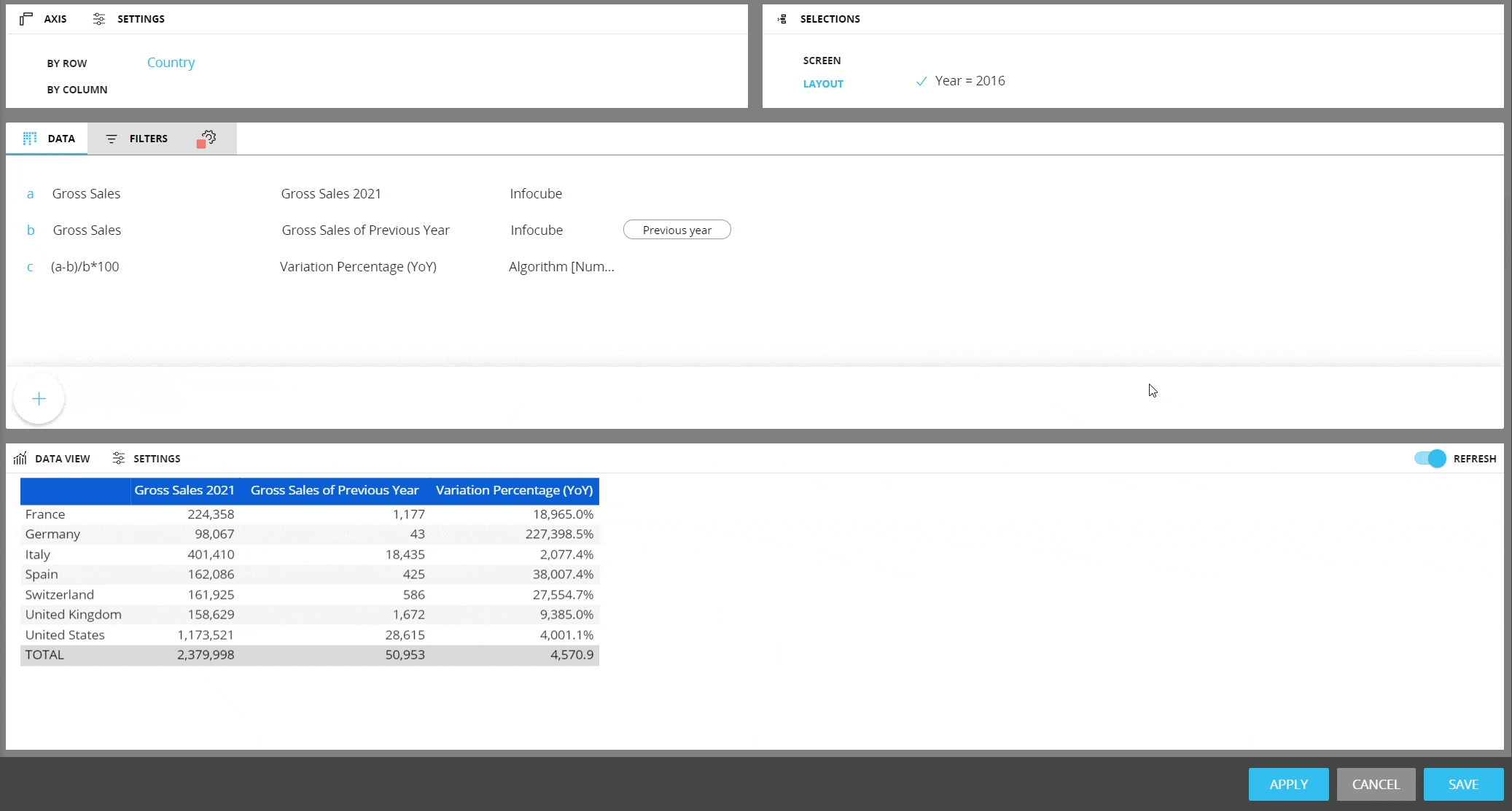The Picture option, (applicable to the objects Data View, Label and Button), allows to display images in the Screen Object. For this to happen, the formula has to return the name of a picture file and the Picture option has to be selected.

Image files must first be uploaded to the Capsule. To upload an image, open the Capsule in Design mode and click on "Images" in the left panel. Once in the Images section, click on "+ ADD IMAGE" and follow the on-screen instructions.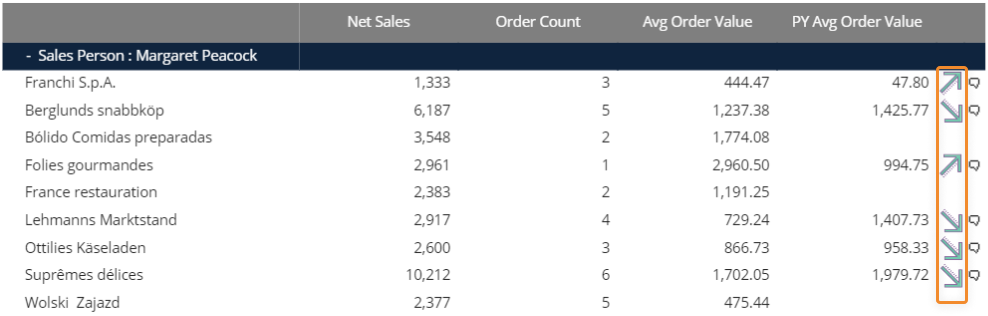Example of images displayed in a Data View

The highlighted images are the result of a picture algorithm using the following formula: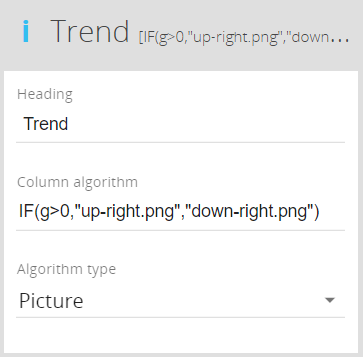In the formula, Block "g" refers to a Block that calculates the variance between the average order value and the previous year average order value. If that value is positive (greater than zero), the up facing arrow is displayed. If that value is negative (less than zero), the down facing arrow is displayed.

Supported file formats are JPG, GIF and PNG. The maximum file size is 500 Kb.

## Supported arithmetic operators

The following table lists all supported arithmetic operators that can be used in formulas.

 Type Operator Meaning Example Arithmetic + Addition a+b - Subtraction a-b / Division a/b * Multiplication a*b % Percentage a/b% is equivalent to a/b*100 a*(1+15%) ^ Exponentiation a^2 is equal to a*a Comparison = Equal to a=b > Greater than a>b < Less than a= Greater than or equal to a>=b <= Less than or equal to a<=b <> Not equal to a<>b Text & Concatenation a&b concatenates two text strings contained in Block "a" and Block "b" a&”myText” concatenates the string contained in Block "a" with "myText". When using this operator, the result of the algorithm is a text string therefore you must select the option Text from the Algorithm type dropdown list. left(text,num_char) Left substring Returns the leftmost characters from a text string.   Left(a,4) returns the first four characters of the text contained in Block "a" right(text,num_char) Right substring Returns the rightmost characters from a text string. Right(a,4) returns the last four characters of the text contained in block "a" mid(text,start_pos,num_char) Substring Returns the specified number of characters from a text string starting from the specified position. Mid(a,2,4) returns the four characters starting from position 2 of the text contained in block "a"

## Supported functions

The following table lists all supported functions that can be used in formulas.

 Function Description Example ABS(number) Returns the absolute value of a number ABS(a-b) AND(logical conditions list) Returns True if all conditions are True, otherwise it returns False AND(a>0,b<100) returns True if the value in Block "a" is greater than zero and the value in Block "b" is less than 100 OR(logical conditions list) Returns True if at least one condition is True. Returns False if all conditions are False OR(a>0,b<100) returns True if the value in Block "a" is greater than zero or if the value in Block "b" is less than 100 IF(condition,true_value,false_value) Returns true_value if the condition is True and false_value if the condition is False The condition can be any logical expression if((a*b)>0,a,c) if(a>100,”Greater”, ”Lower”) if(and(a>0,b>0),a*b,0) Today( ) Returns the current date today()-a returns the number of days between the date in Block "a" and the current date, provided a date Cube is in Block "a" today()+a returns a date resulting from the current date plus the number of days specified in Block "a" dt(column) Returns the column total. To use this function, the Layout must have an entity set by row a/dt(a)*100 rt(column) Returns the row total. To use this function, the Layout must have an entity set by column a/rt(a)*100 gt(column) Returns the grand-total. To use this function, the Layout must have an entity set by row and by column a/gt(a)*100

The above examples use the comma as list separator. The list separator character may vary depending on your computer’s settings.
By default, Board uses the list separator defined under Regional Settings in your computer's Control Panel. The US English version of Board uses a comma (,) for list separator by default, while other international versions may use a semicolon (;).